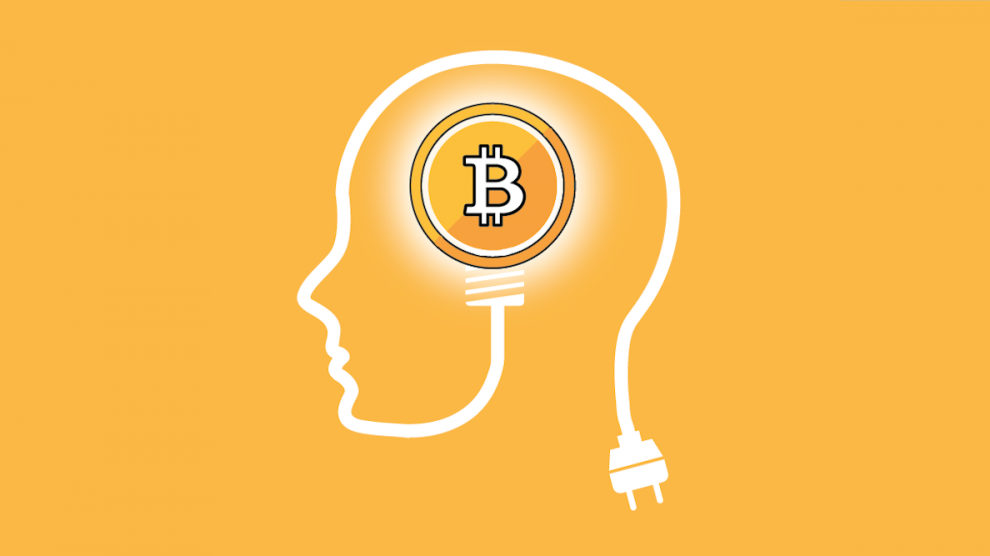# Satoshi Calculates The Probability Of An Bitcoin Network AttackHere it is, folks! The end of the white paper! Let’s see how Satoshi wraps things up.

# Calculations

In this final section of the white paper, Satoshi uses math to prove how difficult it is for a dishonest attacker to double spend transactions on the Bitcoin network.

In order to attack the Bitcoin network, an attacker needs to generate a set of blocks that competes with the longest chain.

If an attacker is able to do this, then he can double-spend his own transaction. In other words, if an attacker already sent a transaction sending bitcoins to someone, then the attacker can later resend the same bitcoins in a new chain that he created and which everyone will consider real.

“Even if this is accomplished, it does not throw the system open to arbitrary changes, such as creating value out of thin air or taking money that never belonged to the attacker. Nodes are not going to accept an invalid transaction as payment, and honest nodes will never accept a block containing them. An attacker can only try to change one of his own transactions to take back money he recently spent.”

Obviously, we do not want this to happen. At any point in time, we can expect attackers like this to exist in the network, and we must have a way to protect against it.

One way to frame this problem, Satoshi says, is to think of it as a “Binomial Random Walk.

“The race between the honest chain and an attacker chain can be characterized as a Binomial Random Walk.”

Every block an attacker produces reduces the deficit. Every block the honest network produces increases the deficit.

Satoshi uses the analogy of a Gambler’s Ruin problem to calculate the probability that an attacker can catch up from a deficit. The more blocks that the attacker has to catch up to, the less probability there is that he can catch up. And this probability increases exponentially with each block.

“Suppose a gambler with unlimited credit starts at a deficit and plays potentially an infinite number of trials to try to reach breakeven.”

What comes next is a lot of fancy math. If you’re a math nerd, you will love this section. If you don’t love math, don’t be intimidated. I’ll explain it at a high-level.

Basically, the math tries to calculate the probability of an attacker being able to catch up when starting from a deficit of “z” blocks. It calculates this probability for various values of “q” (q representing the probability that the attacker can find the next block)

In this case, Satoshi shows the probabilities for q = 0.1 (i.e. 10% probability that an attacker finds the next block) and q = 0.3 (i.e. 30% probability that an attacker finds the next block). You can see from the results above that the probability drops off exponentially each time z increases by 1 (i.e., each time the deficit that the attacker is starting from increases by 1).

When q is 10%, then by the sixth block, there is a 0.02428% probability of an attacker being able to double spend the bitcoins in that block. If q is 30%, then by the tenth block, there is a 4.1% probability of an attacker being able to double spend the bitcoins in that block.

Next, Satoshi solves for the probability of an attacker catching up being less than 0.1%. In other words, if we want the probability to be less than 0.1%, what would be if we assume various values of q?

If we want to have a probability of less than 0.1% of an attacker catching up, then at q = 10%, we need to wait 5 blocks. At q = 15%, we need to wait 8 blocks. At q = 20%, we need to wait 11 blocks. And so on.

This is why when we send a Bitcoin transaction, we typically wait “six confirmations” before the transaction is considered valid. We could wait less blocks, but then there is less of a guarantee that the transaction can’t be double spent.

With that, we are done!

Wow, can you believe it?!?!? Congratulations! You just read the Bitcoin whitepaper, and you now understand every single line of it. Go brag to all your friends! 🥳#### Nicolas van Saberhagen

We created Cryptonote algorithm that has been used in creation of Monero.